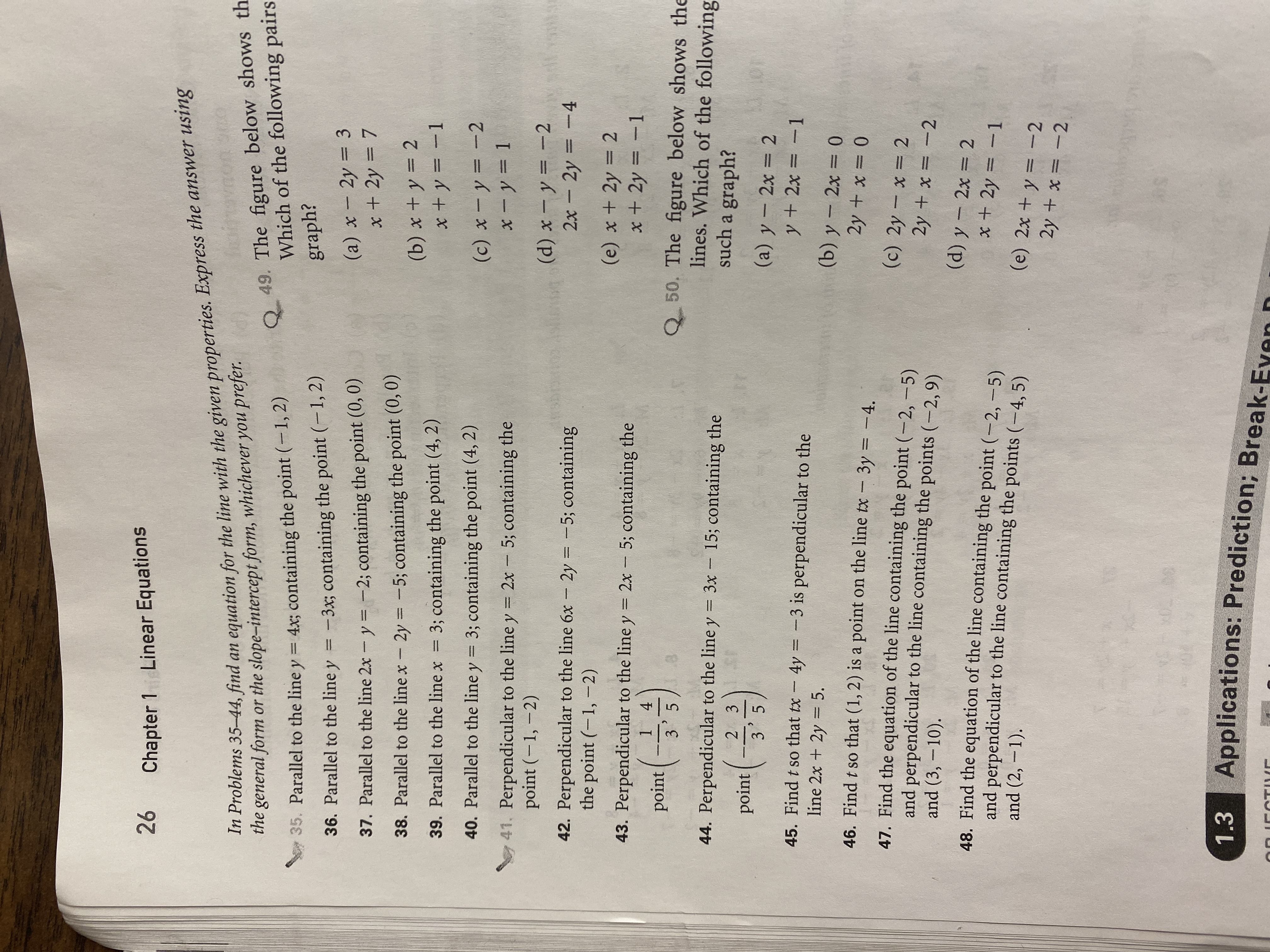# 26Linear EquationsChapter 1m Problems 35–44, find an equation for the line with the given properties. Express the answer usingthe general form or the slope-intercept form, whichever you prefer.fasinavnorQ 49. The figure below shows thWhich of the following pairs35. Parallel to the line y = 4x; containing the point (-1, 2)%3Dgraph?-3x; containing the point (-1, 2)36. Parallel to the line y(a) x – 2y = 3x + 2y = 737. Parallel to the line 2x - y = -2; containing the point (0, 0)38. Parallel to the line x- 2y = -5; containing the point (0, 0)%3D(b) x + y = 2x +y = -13; containing the point (4, 2)39. Parallel to the line x%3D40. Parallel to the line y = 3; containing the point (4, 2)%3D(c) x -y = -2x - y = 141. Perpendicular to the line y = 2x - 5; containing thepoint (-1, –2)%3D(d) x – y = -22x – 2y = -442. Perpendicular to the line 6x - 2y = -5; containingthe point (-1,-2)(e) x + 2y = 2x + 2y = -1%3D43. Perpendicular to the line y = 2x - 5; containing the|3D%3D1point4Q 50. The figure below shows thelines. Which of the followingsuch a graph?3 544. Perpendicular to the line y = 3x - 15; containing the%3D2 3point3 5(a) y – 2x = 2y + 2x = -1%3D45. Find t so that tx - 4y = -3 is perpendicular to theline 2x + 2y = 5.%3D(b) y - 2x = 046. Find t so that (1, 2) is a point on the line tx - 3y = -4.2y + x = 0%3D47. Find the equation of the line containing the point (-2, -5)and perpendicular to the line containing the points (-2,9)and (3, -10).(c) 2y - x = 22y + x = -2(d) y - 2x = 248. Find the equation of the line containing the point (-2,-5)and perpendicular to the line containing the points (-4, 5)and (2, -1).x + 2y = -1%3D(e) 2x + y = -22y + x = -2Applications: Prediction; Break-Even D1.3

Question
14 views

I need some help solving number 45 pleasehelp_outlineImage Transcriptionclose26 Linear Equations Chapter 1 m Problems 35–44, find an equation for the line with the given properties. Express the answer using the general form or the slope-intercept form, whichever you prefer. fasinavnor Q 49. The figure below shows th Which of the following pairs 35. Parallel to the line y = 4x; containing the point (-1, 2) %3D graph? -3x; containing the point (-1, 2) 36. Parallel to the line y (a) x – 2y = 3 x + 2y = 7 37. Parallel to the line 2x - y = -2; containing the point (0, 0) 38. Parallel to the line x- 2y = -5; containing the point (0, 0) %3D (b) x + y = 2 x +y = -1 3; containing the point (4, 2) 39. Parallel to the line x %3D 40. Parallel to the line y = 3; containing the point (4, 2) %3D (c) x -y = -2 x - y = 1 41. Perpendicular to the line y = 2x - 5; containing the point (-1, –2) %3D (d) x – y = -2 2x – 2y = -4 42. Perpendicular to the line 6x - 2y = -5; containing the point (-1,-2) (e) x + 2y = 2 x + 2y = -1 %3D 43. Perpendicular to the line y = 2x - 5; containing the |3D %3D 1 point 4 Q 50. The figure below shows the lines. Which of the following such a graph? 3 5 44. Perpendicular to the line y = 3x - 15; containing the %3D 2 3 point 3 5 (a) y – 2x = 2 y + 2x = -1 %3D 45. Find t so that tx - 4y = -3 is perpendicular to the line 2x + 2y = 5. %3D (b) y - 2x = 0 46. Find t so that (1, 2) is a point on the line tx - 3y = -4. 2y + x = 0 %3D 47. Find the equation of the line containing the point (-2, -5) and perpendicular to the line containing the points (-2,9) and (3, -10). (c) 2y - x = 2 2y + x = -2 (d) y - 2x = 2 48. Find the equation of the line containing the point (-2,-5) and perpendicular to the line containing the points (-4, 5) and (2, -1). x + 2y = -1 %3D (e) 2x + y = -2 2y + x = -2 Applications: Prediction; Break-Even D 1.3 fullscreen
check_circle

Step 1

Given:

Step 2

Calculation:

Step 3

### Want to see the full answer?

See Solution

#### Want to see this answer and more?

Solutions are written by subject experts who are available 24/7. Questions are typically answered within 1 hour.*

See Solution
*Response times may vary by subject and question.
Tagged in
MathAlgebra

### Other